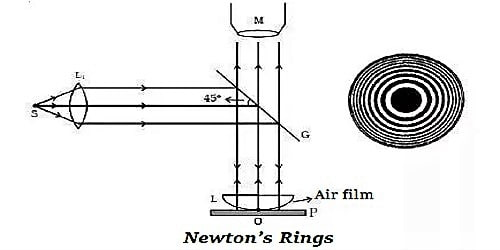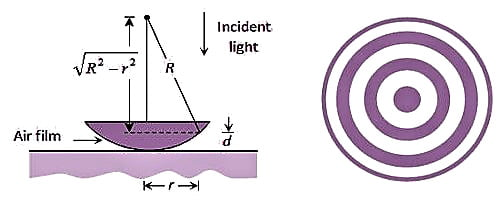# Describe Applications of Newton’s Rings

An essential application of interference in thin films is the arrangement of Newton’s rings. Newton’s Rings are rings caused by the slim film effect. You can use them to verify the thickness of a surface and to test out whether a surface is a uniform.

Applications of Newton’s rings

(i) Using the method of Newton’s rings, the wavelength of a given monochromatic source of light can be determined. The radius of nth dark ring and (n+m)the dark ring are given by

rn2 = nRλ and r2n+m = (n+m) Rλ

rn+m2 – rn2 = mRλ

so, λ = [rn+m2 – rn2] / mR

Knowing rn+m, rn, and R, the wavelength can be calculated.(ii) Using Newton’s rings, the refractive index of a liquid can be calculated. Let λa and λm represent the wavelength of light in air and in medium (liquid). If rn is the radius of the nth dark ring in the air and if rn is the radius of the nth dark ring in liquid, then

rn2 = nRλa

r`n2 = nRλm = nRλa

so, μ = rn2/ r`n2